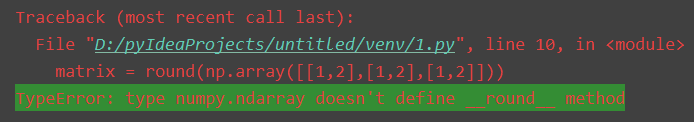# How to Solve TypeError: type numpy.ndarray doesn‘t define __round__ method

Pycharm Warning TypeError: type numpy.ndarray doesn’t define round method

1.the problem
`matrix = round(np.array([[1.5,2.3],[1,2],[1,2]]))`
The error interfacetype is wrong: the type numpy.ndarray does not define the __round__ method

2.solve
Change the round method to np.round
code show as below:

`matrix = np.round(np.array([[1.5,2.3],[1,2],[1,2]]))`